## Friday, January 04, 2019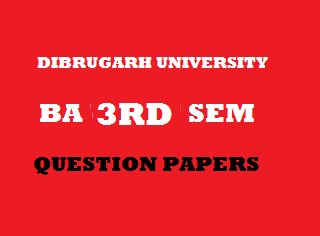2014
(November)
MATHEMATICS
(Major)
Course: 302
(Coordinate Geometry and Algebra - I)
Full Marks: 80
Pass Marks: 32
Time: 3 hours
The figures in the margin indicate full marks for the questions
GROUP – A
(Coordinate Geometry)
SECTION – I
(2-Dimension)
(Marks: 27)

1. (a) Find out the angle between the pair of lines represented by the equation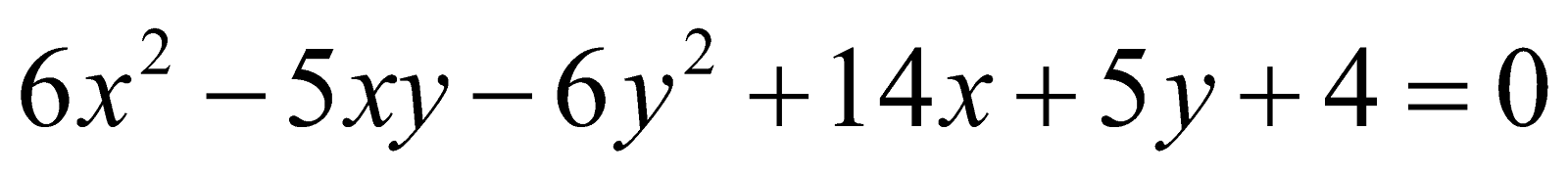1
(b) Choose a new origin (h, k), without changing the directions of the axes such that the equation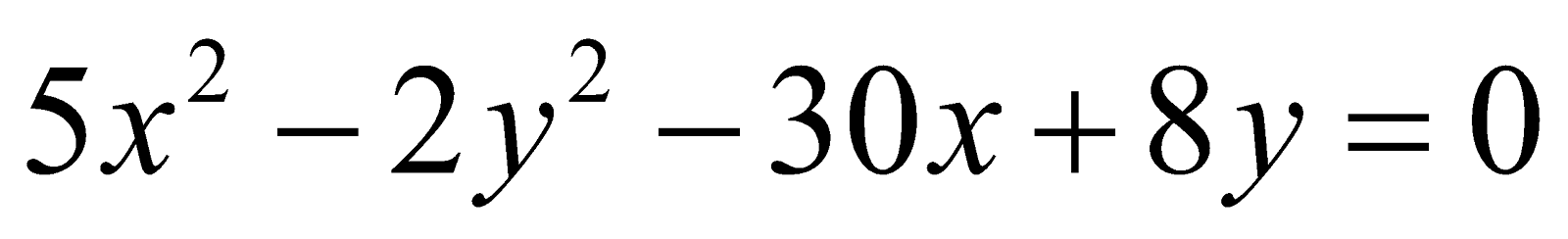may reduce to the form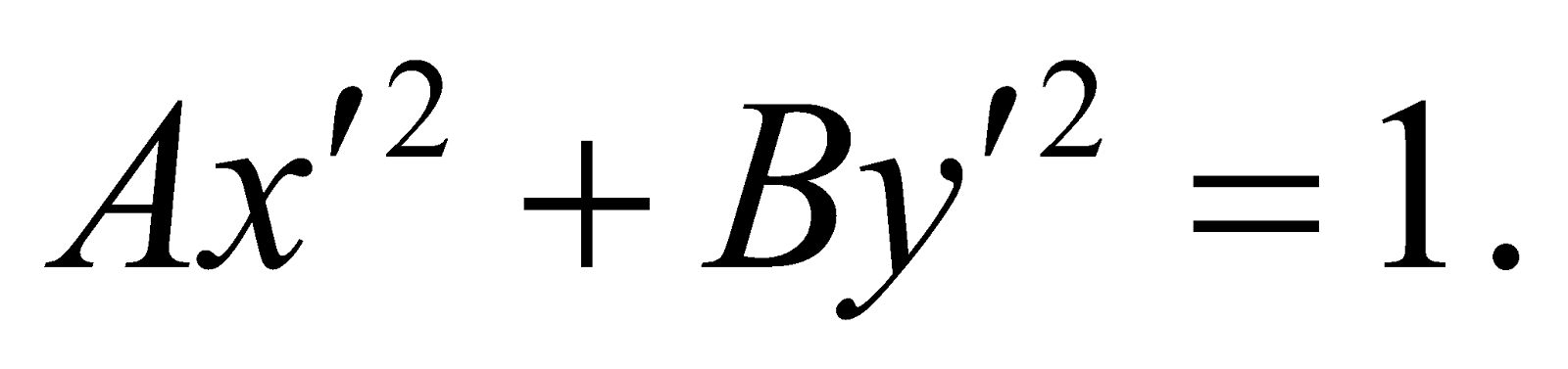2
(c) Transform the equation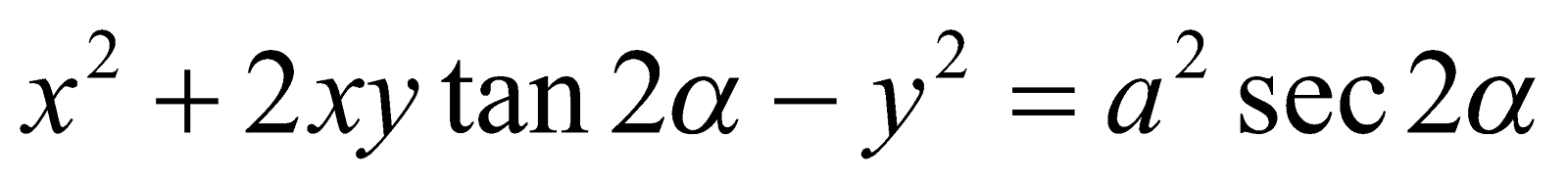To rectangular axes inclined at angle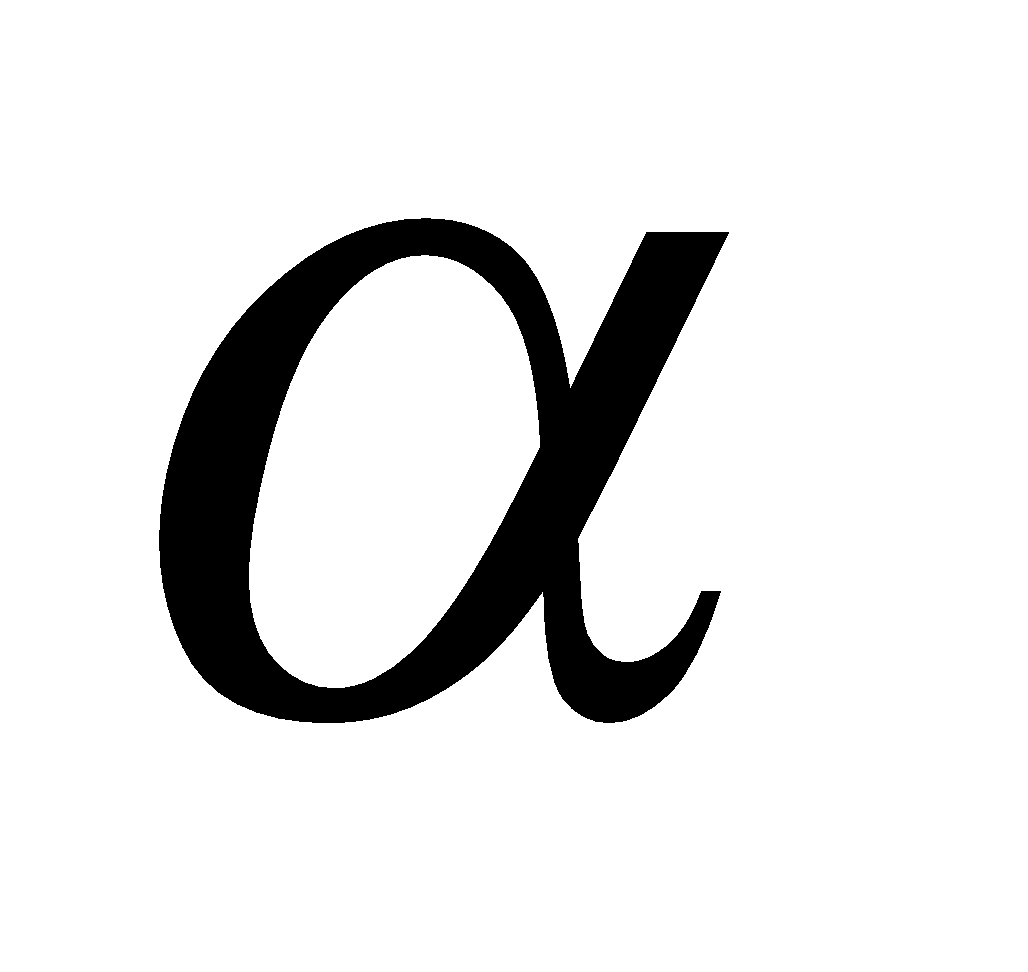to the old rectangular axes. 3
Or
Find the angle through which the axes be rotated so that the expression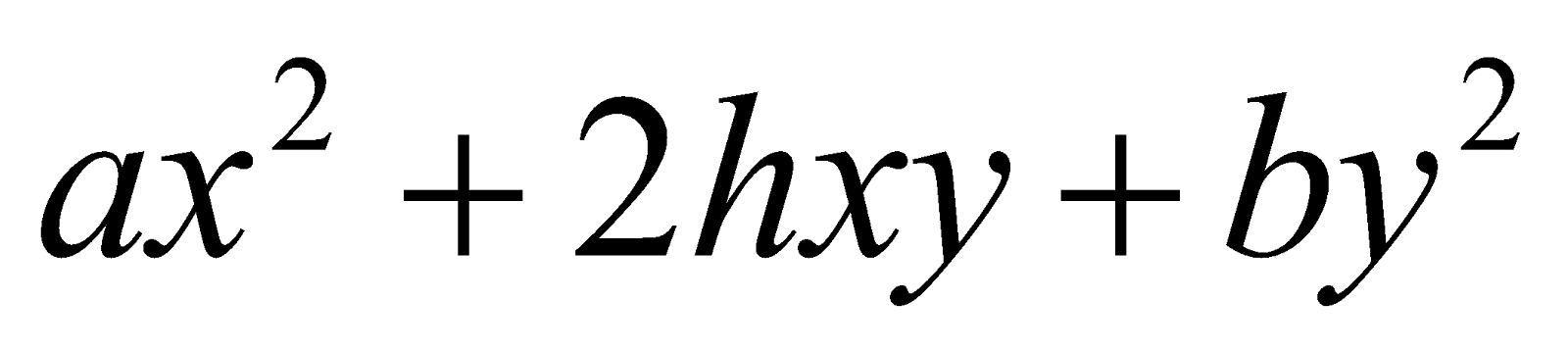may become of the form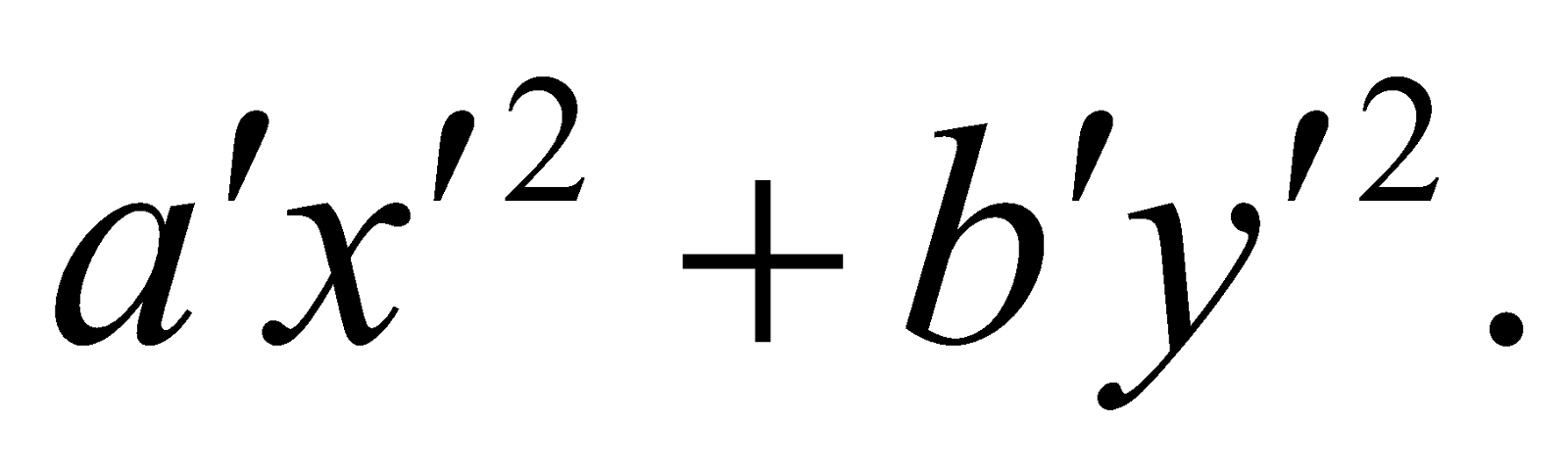2. (a) Find the value of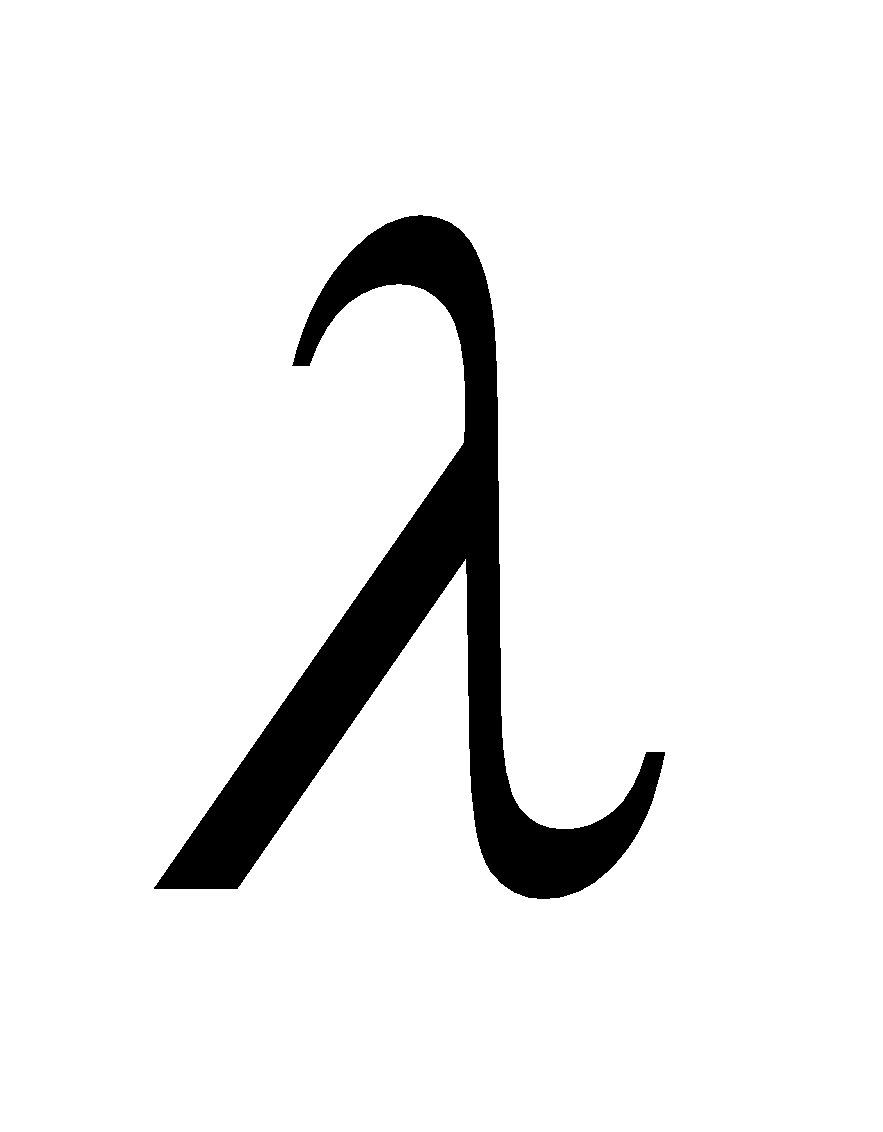so that the equation 3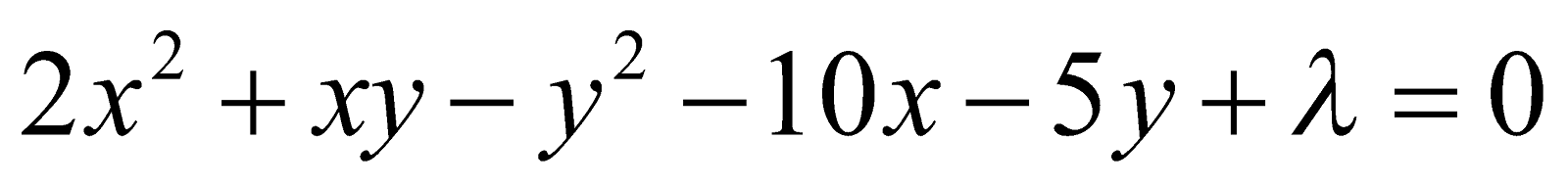may represent a pair of lines.
(b) Find the equation of the pair of lines through the origin which represents the lines perpendicular to the pair of lines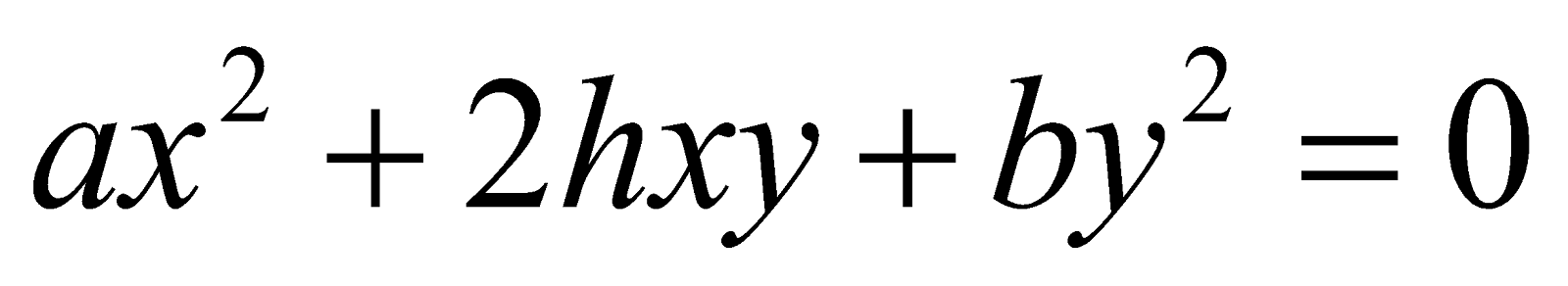. 3
Or
Show that the angle between one of the lines given byand one of the lines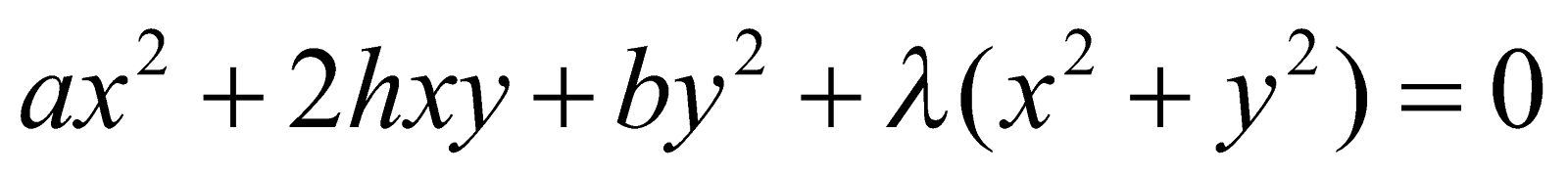is equal to the angle between the other two lines of the systems.
(c) Prove that the straight lines represented by the equation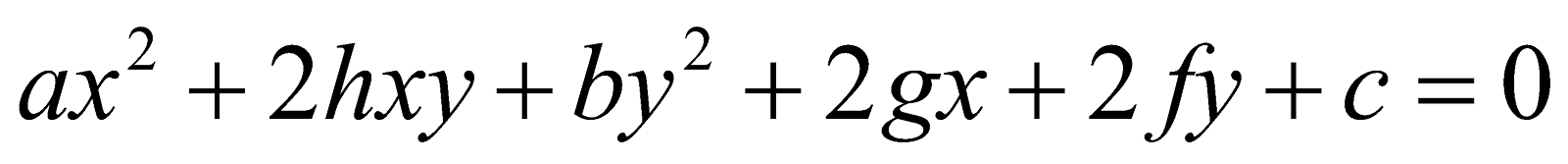Will be equidistant from the origin if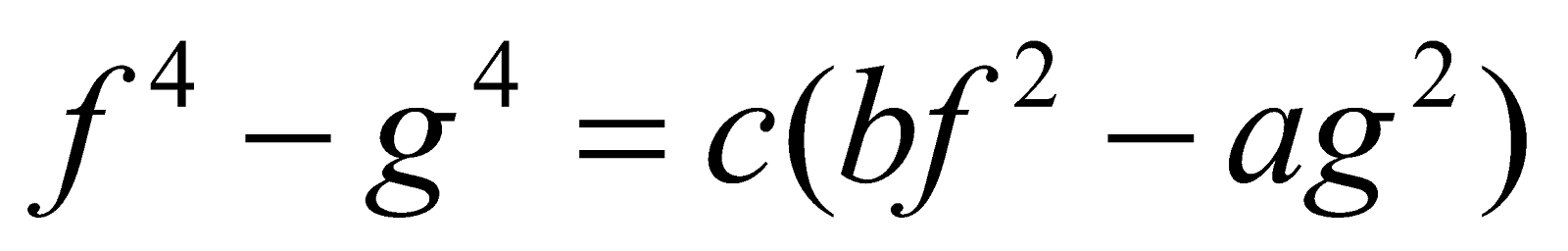5
Or
Ifrepresents a pair of lines, prove that the area of the triangle formed by these lines and the x-axis is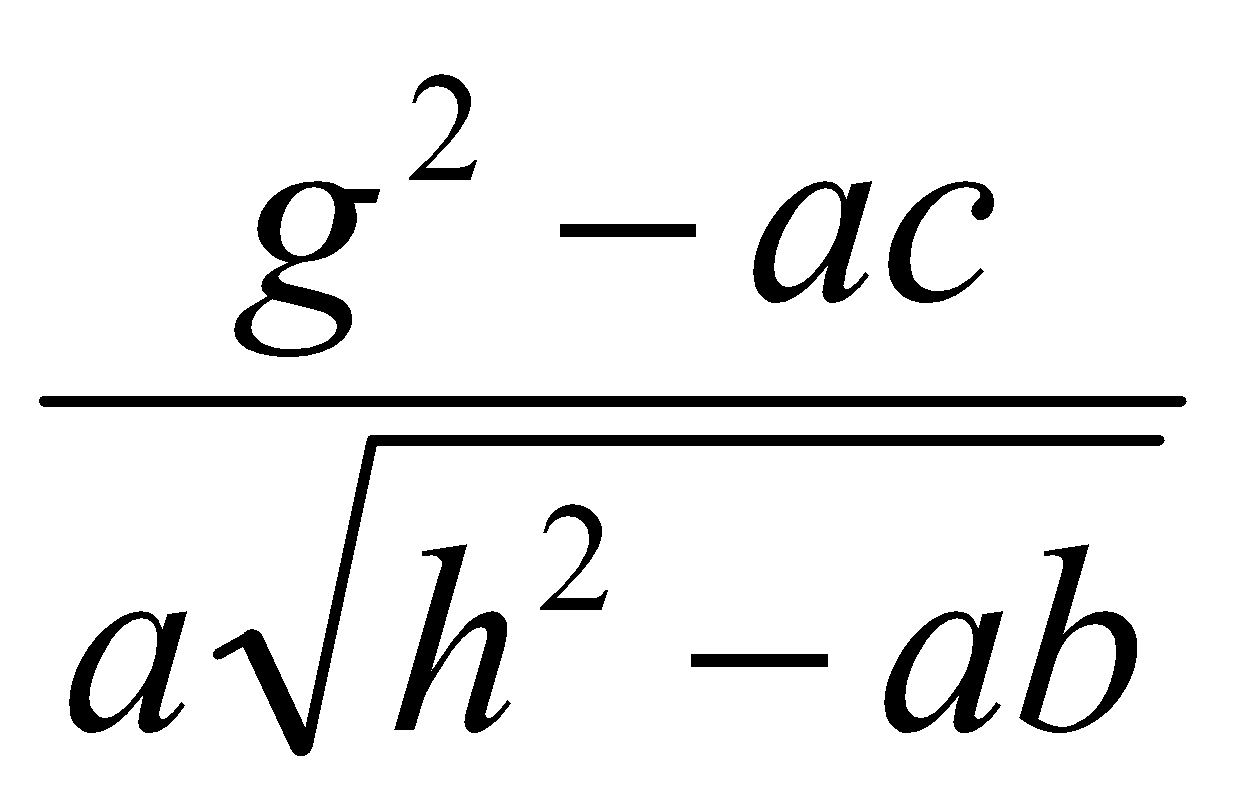3. (a) State True or False: 1
When the focus lies on the directrix, the conic section is a pair of lines.
(b) Find the equation of the chord of contact of tangents from a point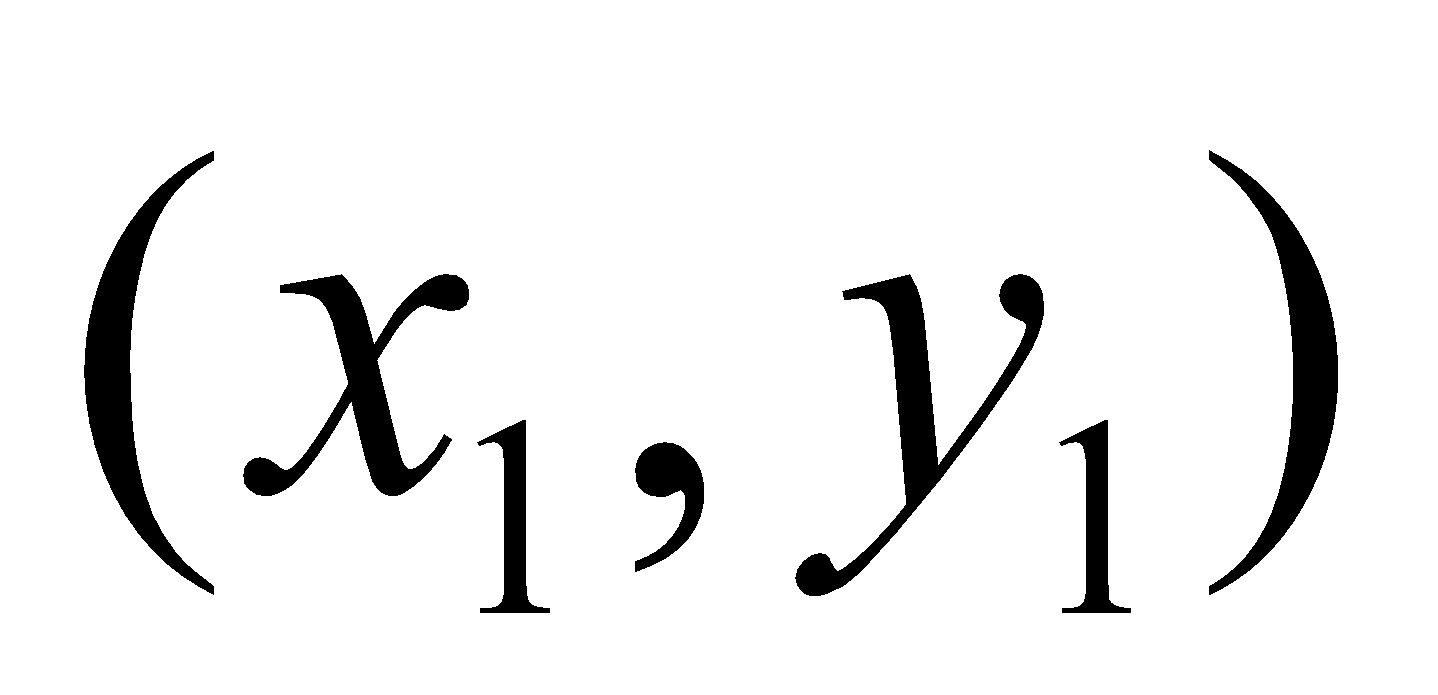to the conic 4Or
Find the condition that the pair of lines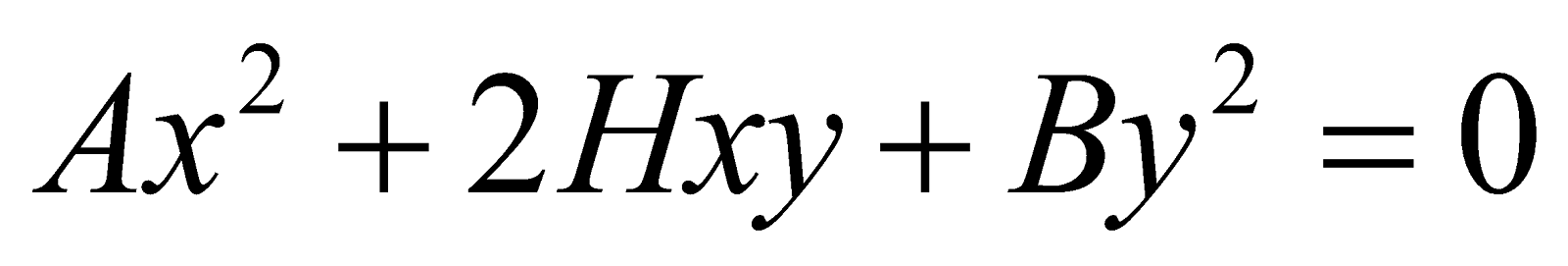may be conjugate diameters of the conic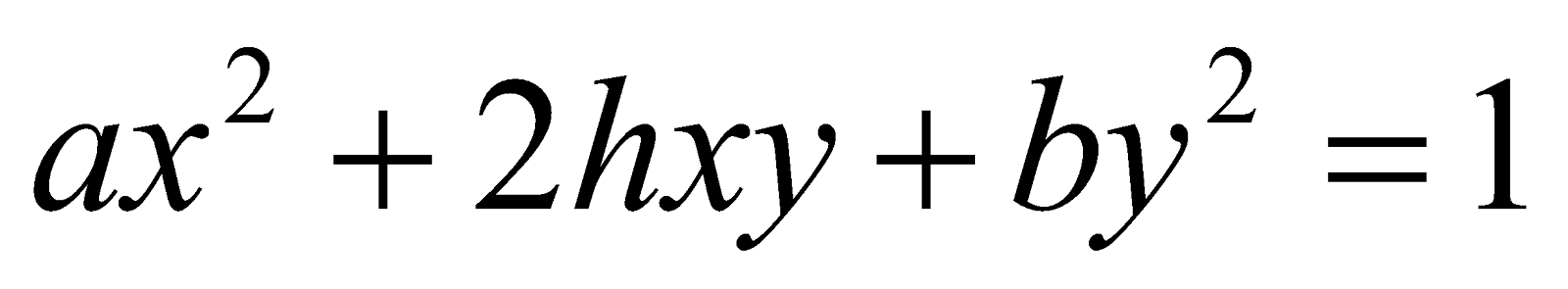(c) Reduce the following equation to the standard form: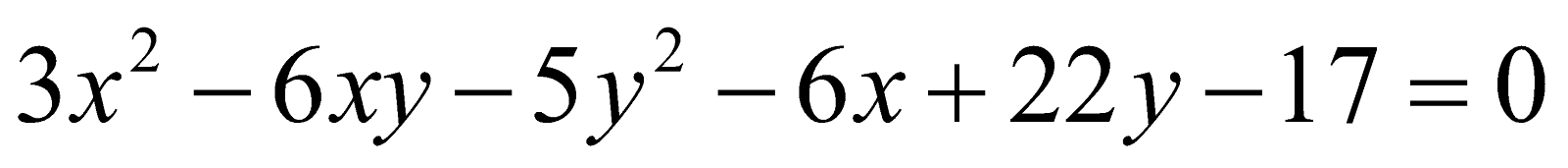5
Or
Prove that every Cartesian equation of the second degree, i.e.represents a conic.

SECTION – II
(3-Dimension)
(Marks: 18)
4. (a) Write the direction cosines of the line joining the origin and the point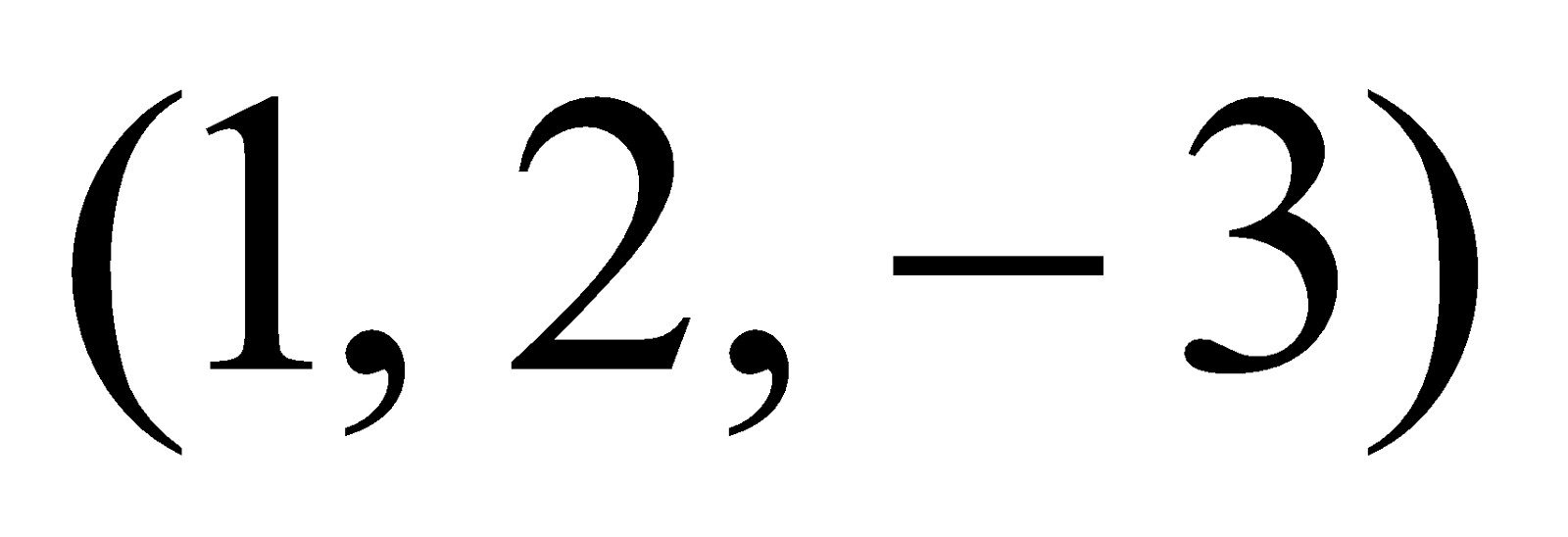. 1
(b) Find the equation of the plane which passes through the intersection of the planes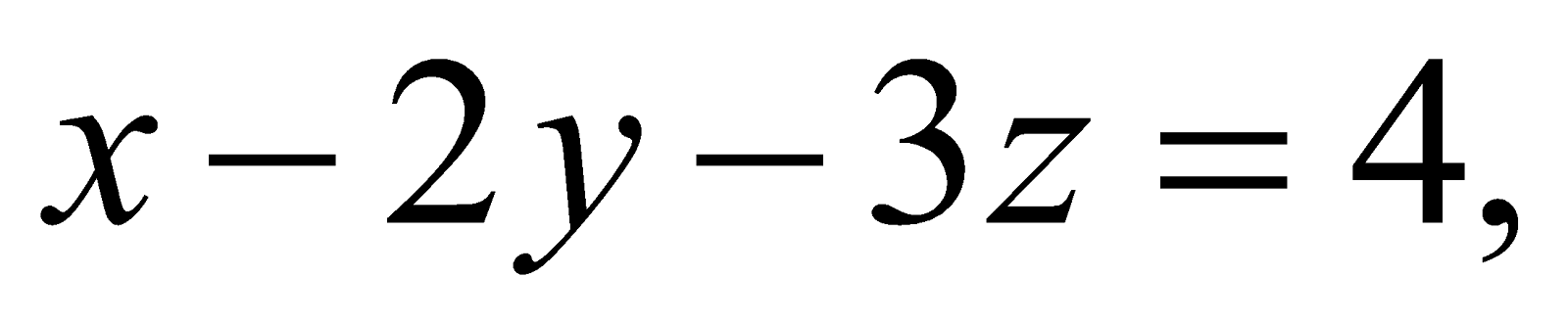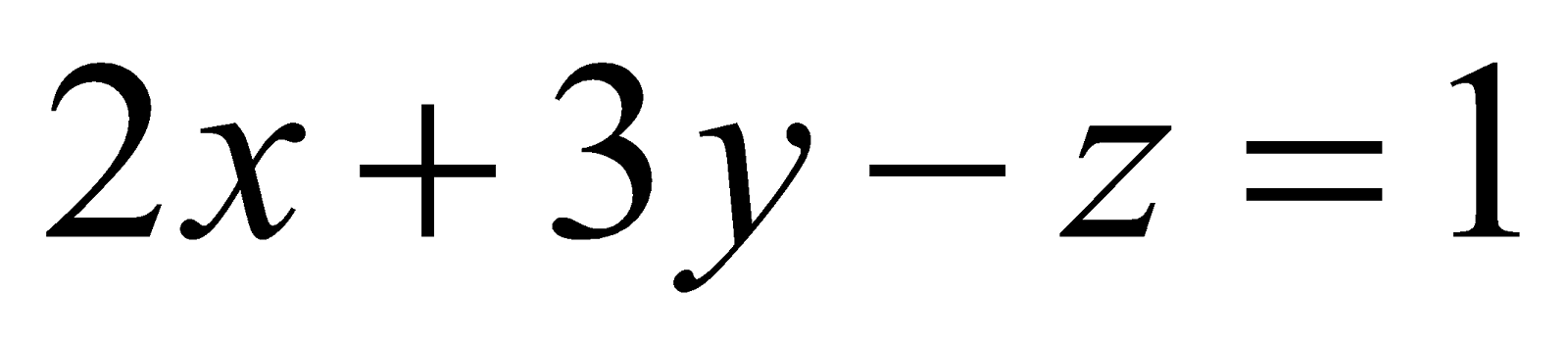and is perpendicular to the plane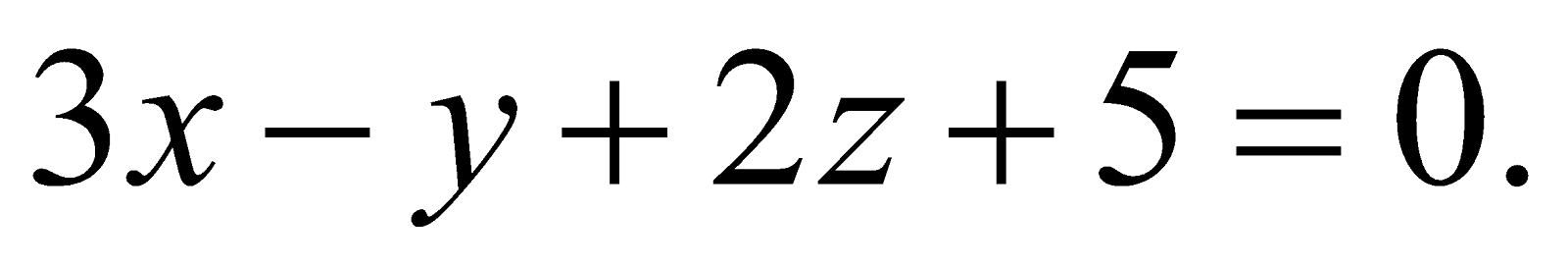4
(c) Find the distance of the point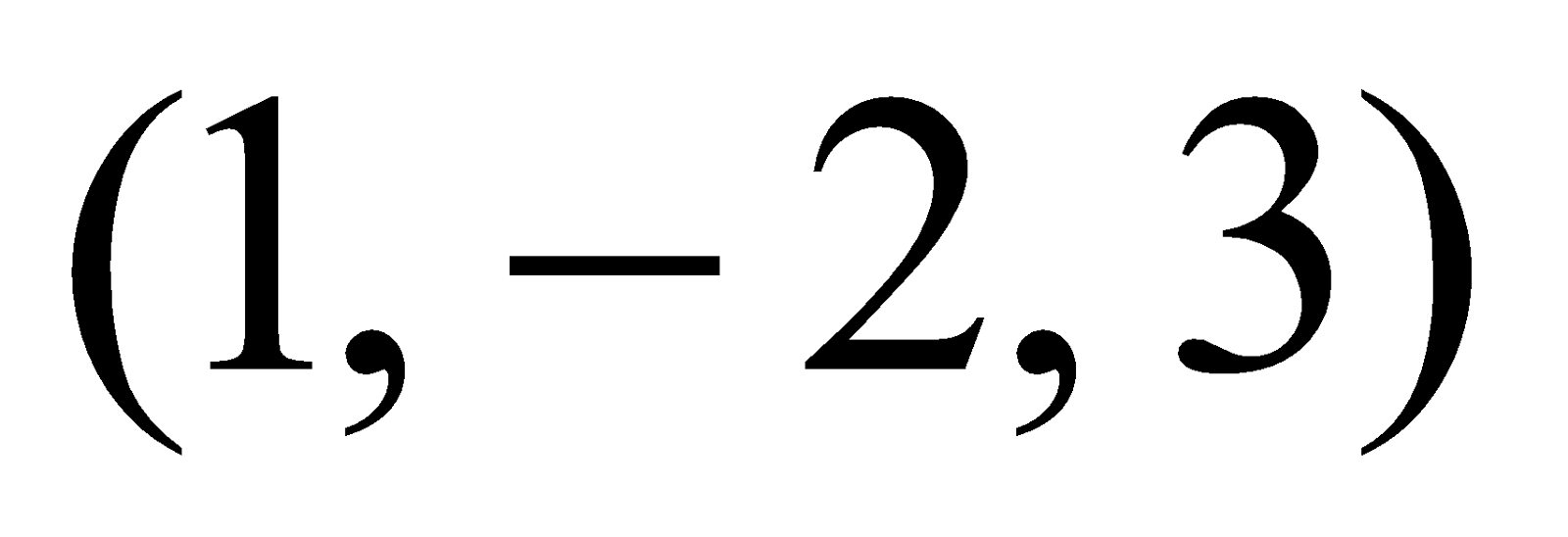from the planemeasured parallel to the line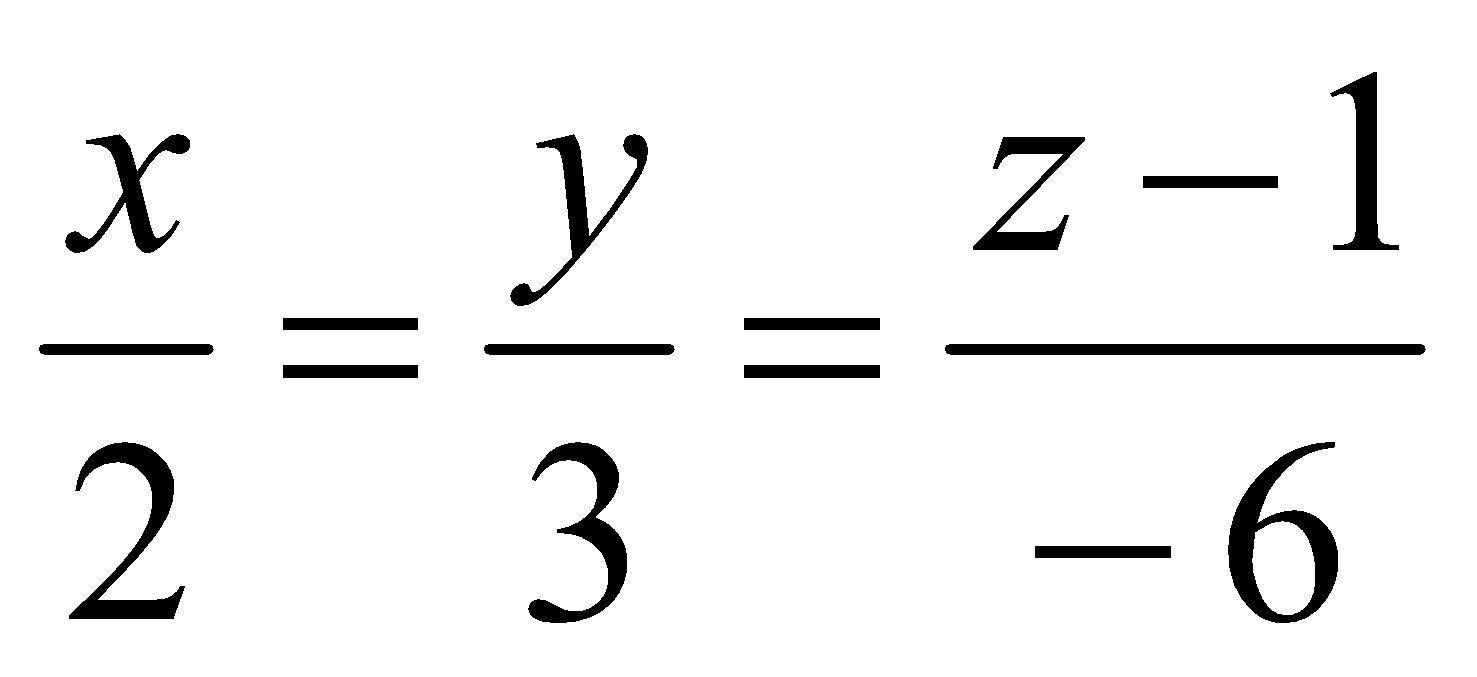5
Or
Show that the lines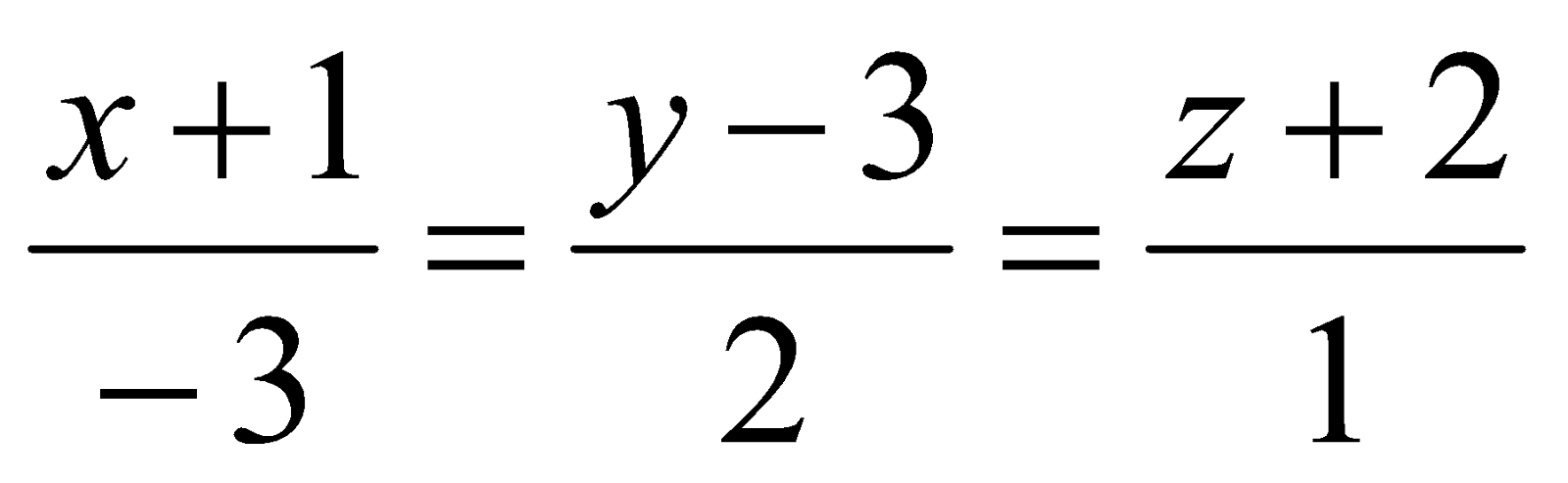and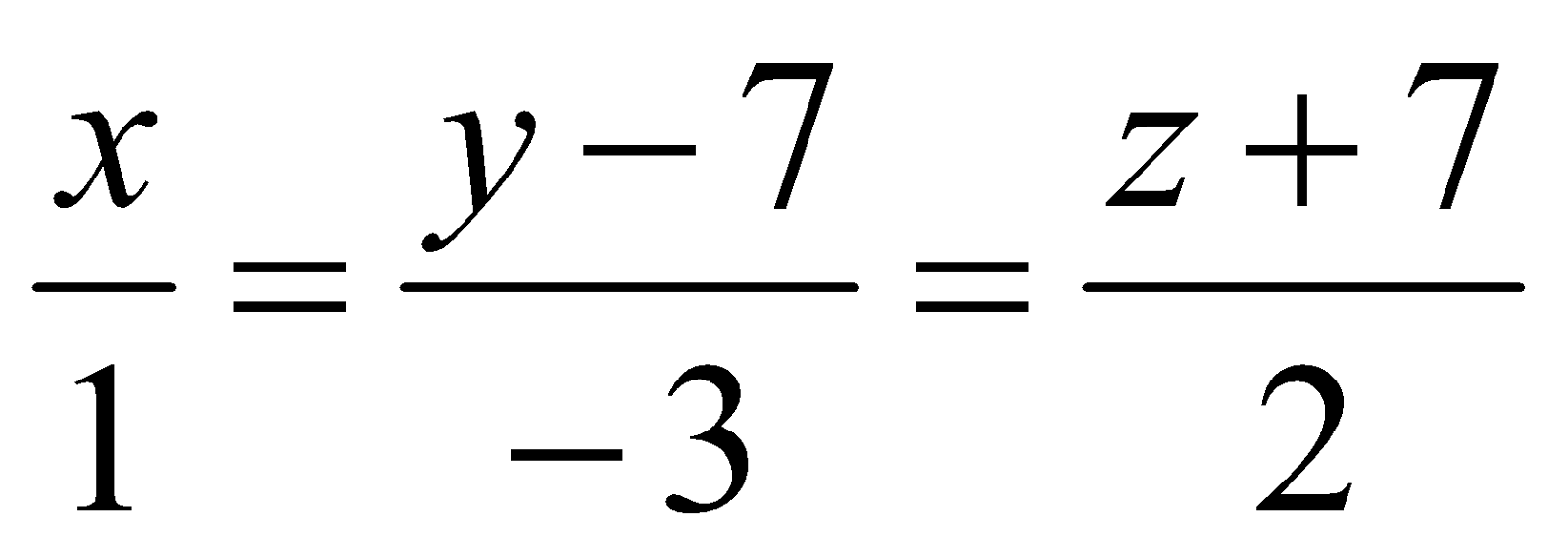intersect. Find the coordinates of the point of intersection.
5. (a) What is the shortest distance between two intersecting straight lines? 1
(b) Find the shortest distance between the line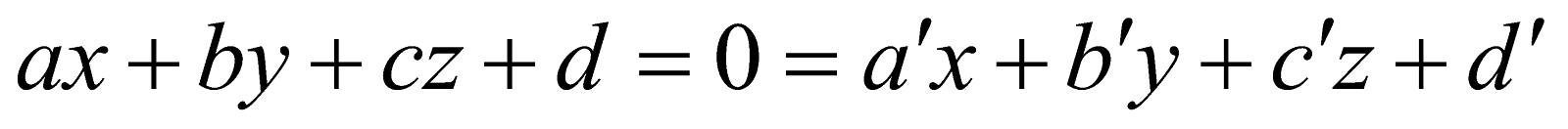2
and the z-axis.
(c) Find the length and the equations of the shortest distance between the lines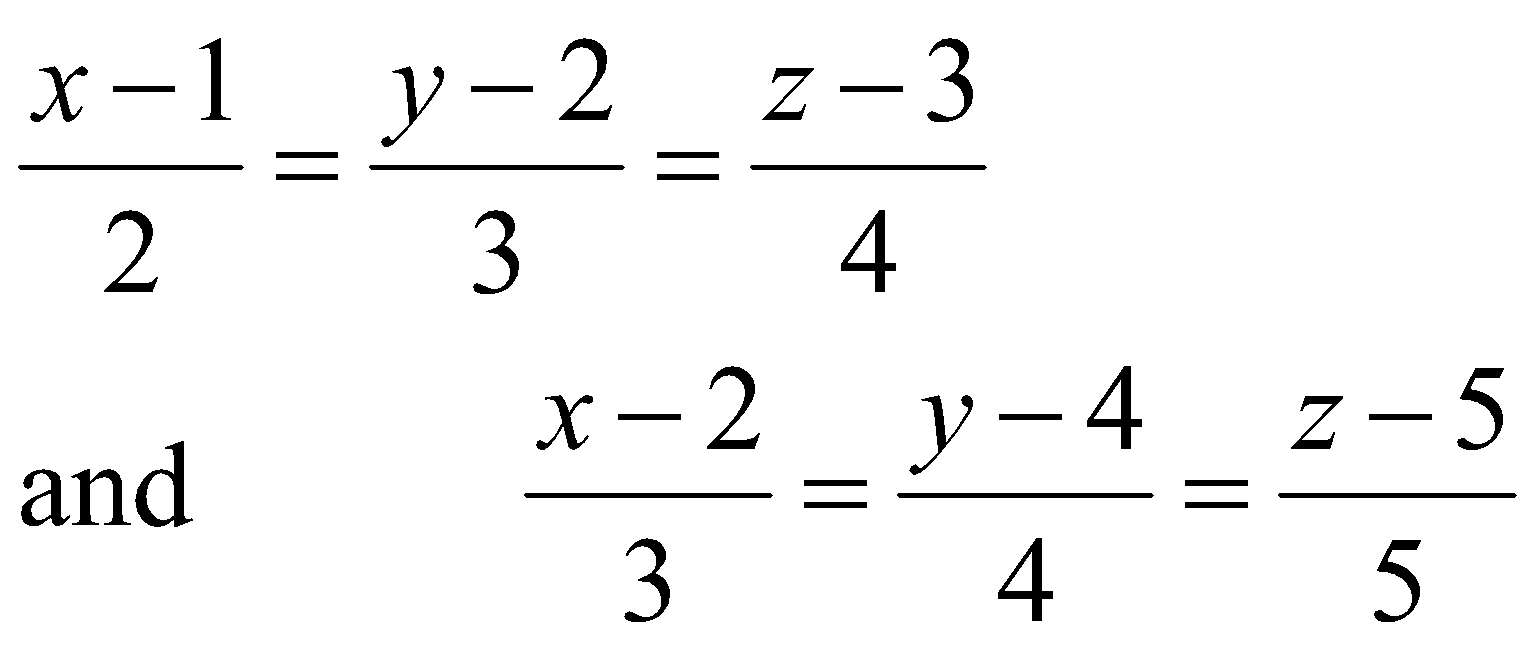5
Or
Prove that the shortest distance between the lines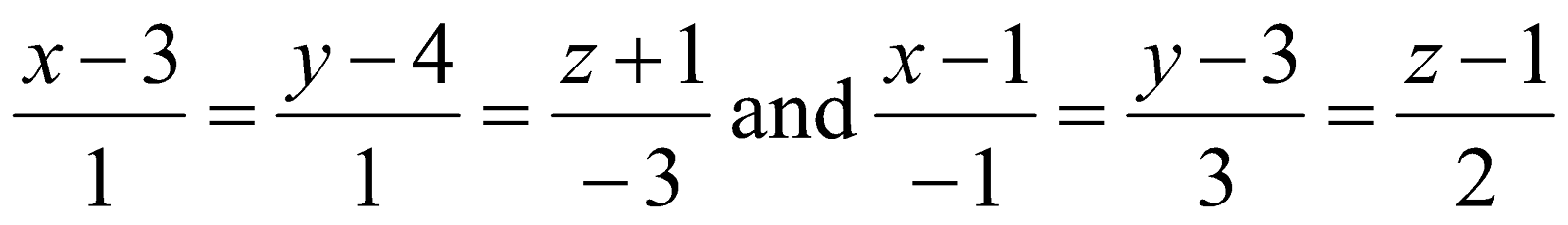is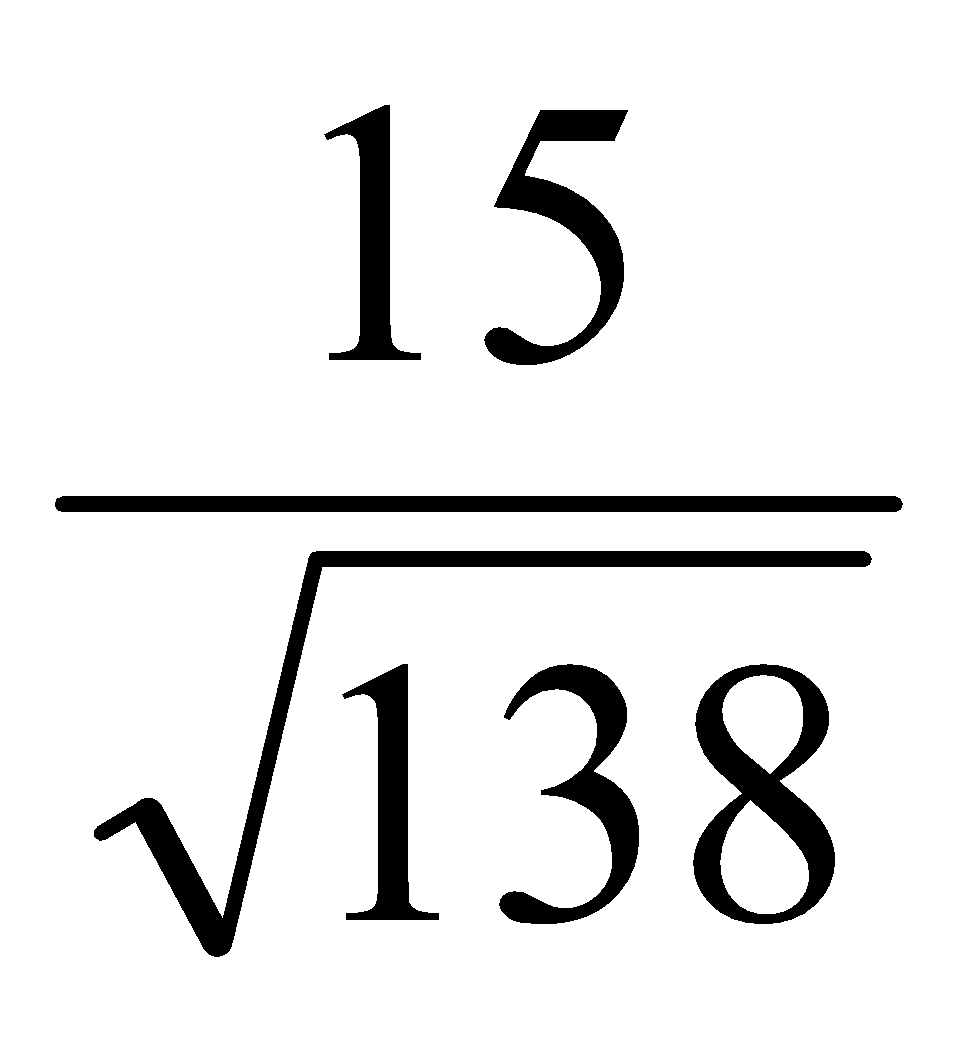and the equation of shortest distance are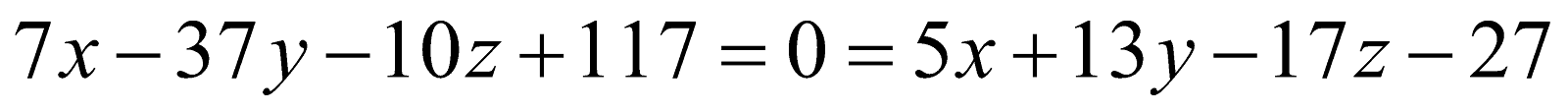GROUP – B
(Algebra – I)
(Marks: 35)

6. (a) If a set A has n members, then state the number of binary compositions on A. 1
(b) The set of integers, with respect to usual multiplication does not form a group. Justify it. 2
(c) State True or False: A group of prime order is Abelian. 1
(d) Answer any two questions: 3x2=6
1. Show that the centre of a group G is a subgroup of G.
2. If G is a group is which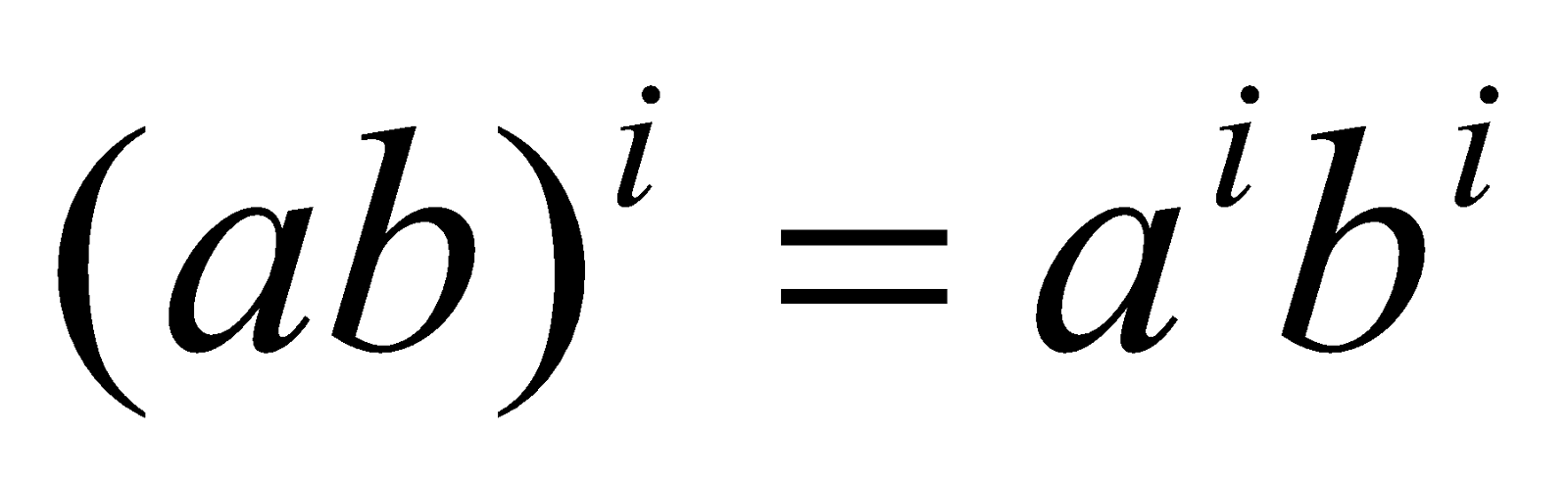for three consecutive integers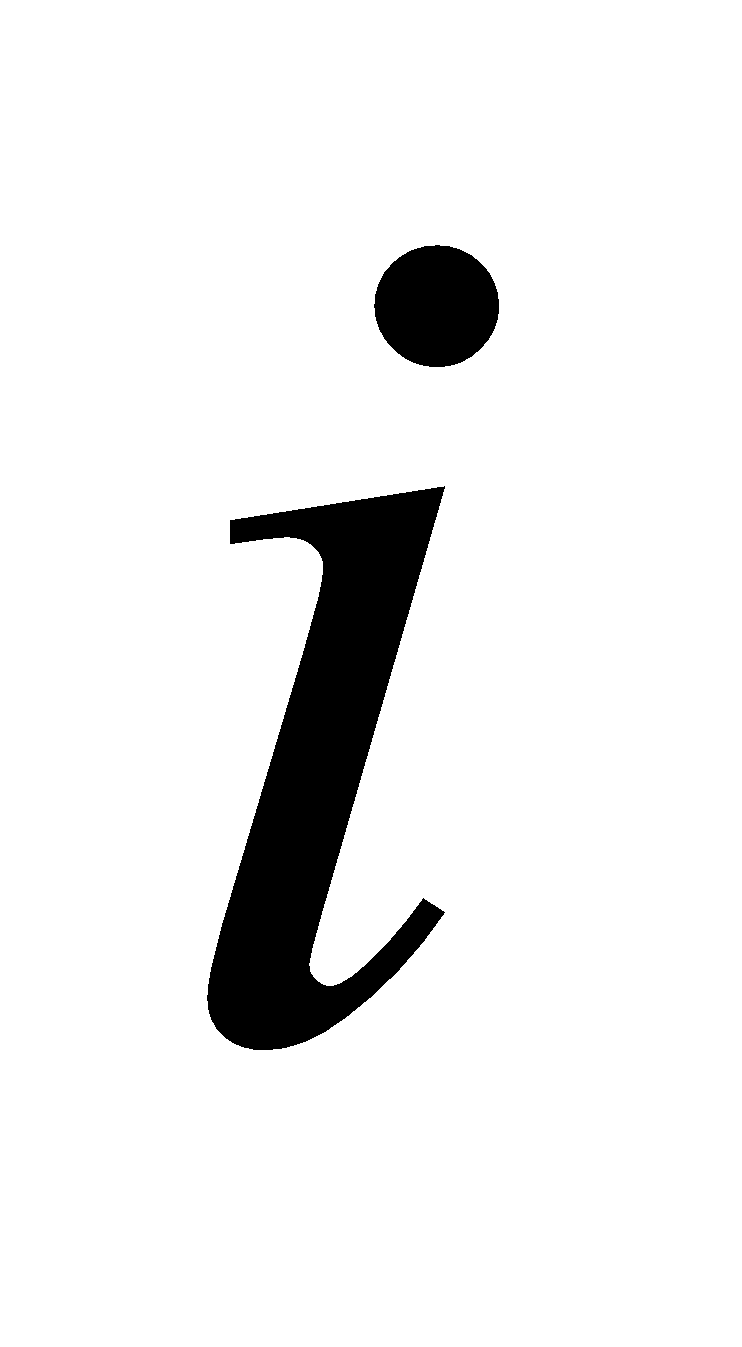and any a, b in G, then show that G is Abelian.
3. Prove that an infinite cyclic group has precisely two generators.
7. Answer any two questions: 5x2=10
1. Let G be a group. Suppose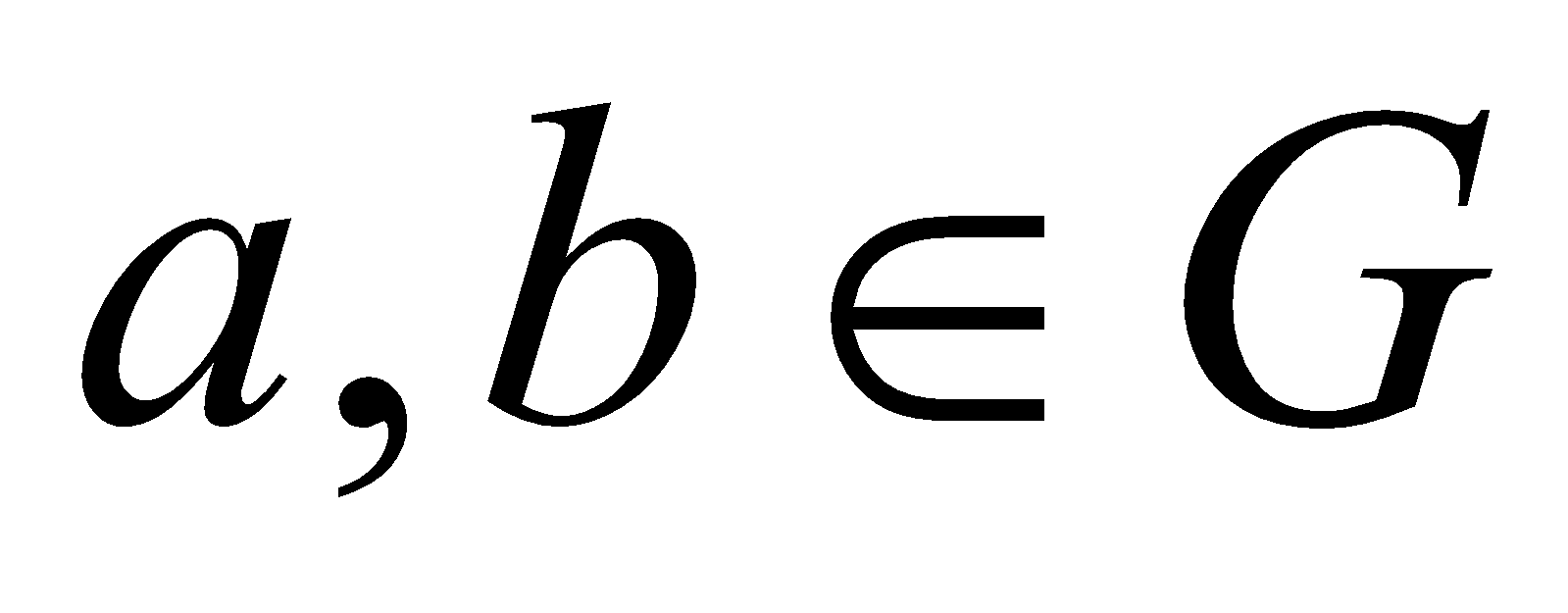such that –
1.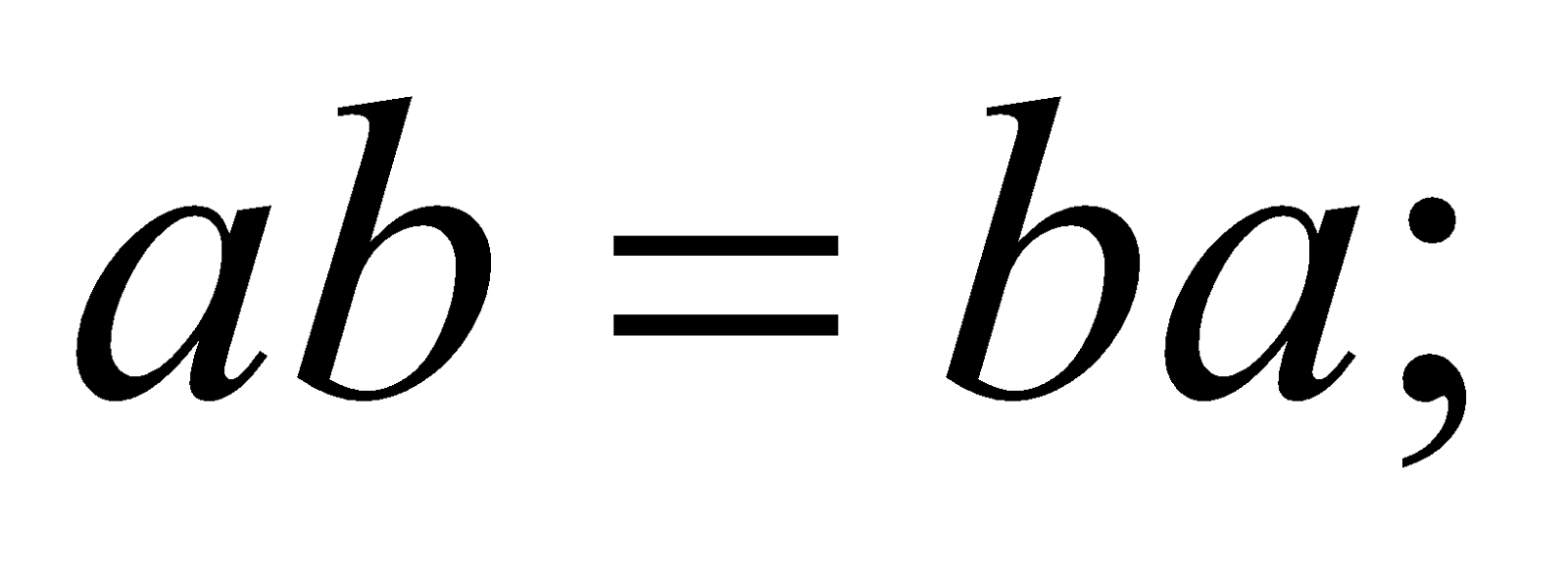2.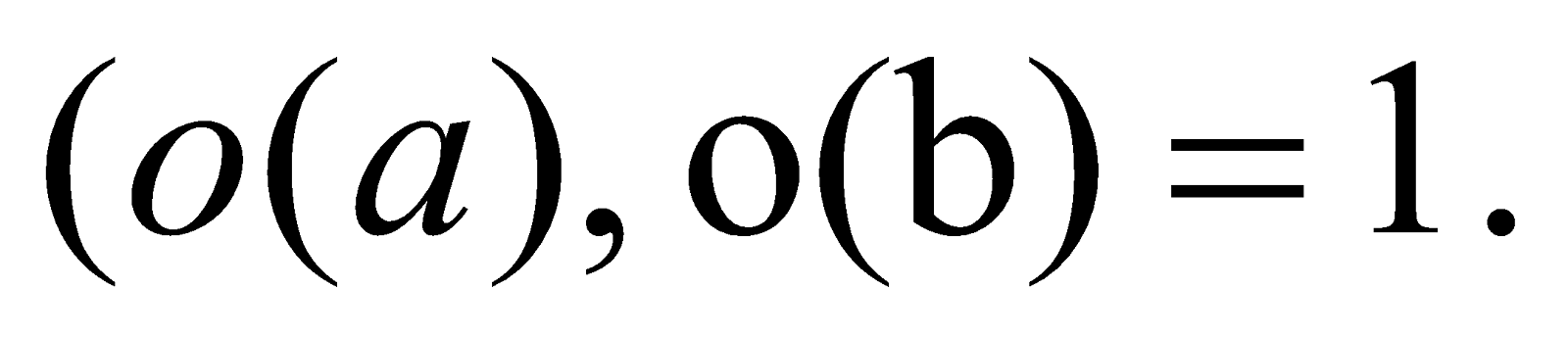3.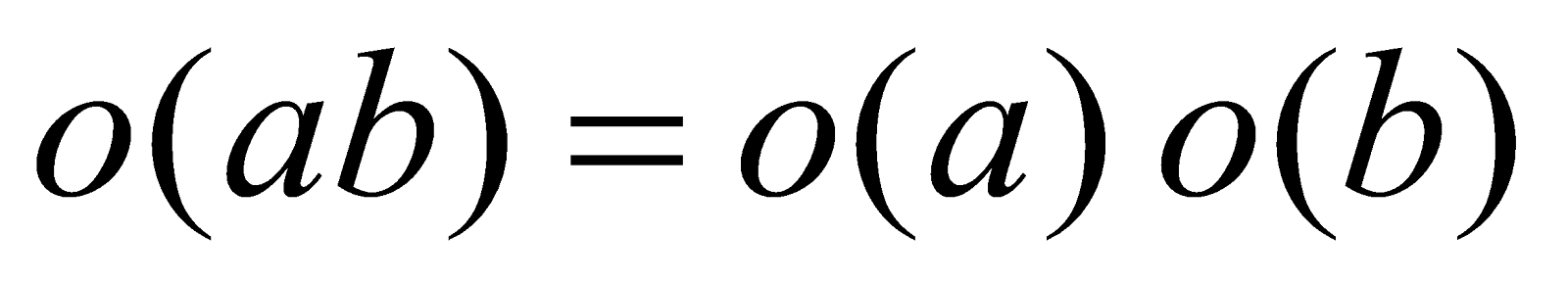.
1. If H and K are finite subgroups of a group G, then prove that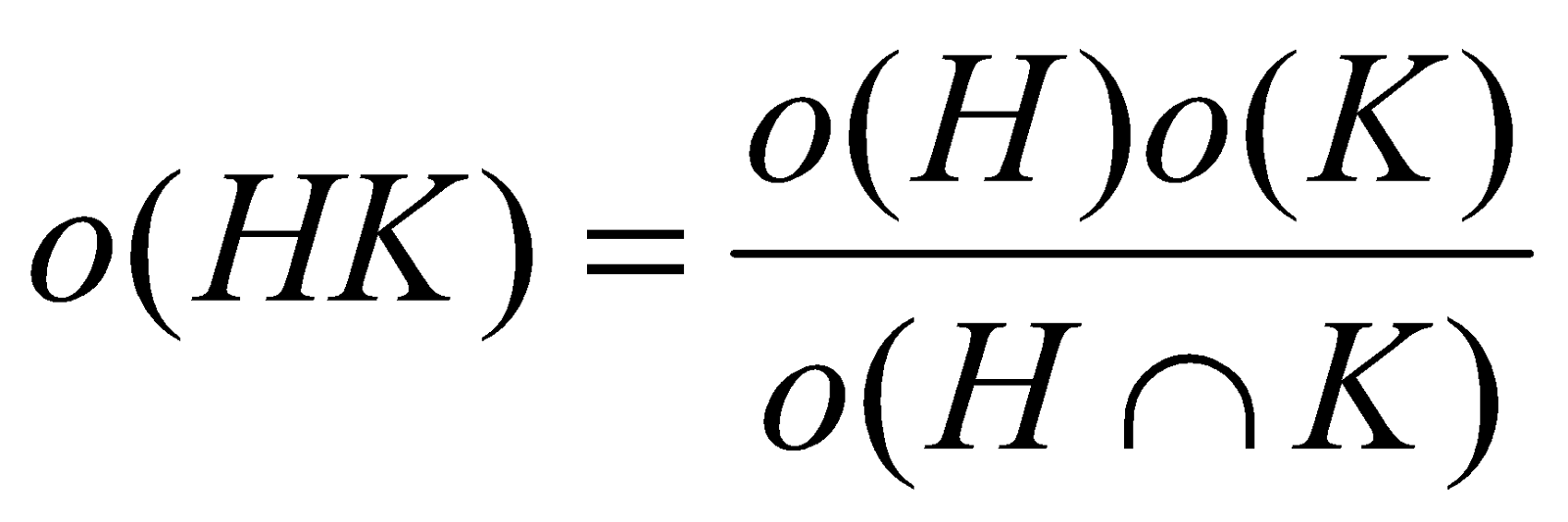1. If a group has finite number of subgroups, then show that it is a finite group.
8. (a) Define Kernel of a group homomorphism. 1
(b) Prove that a subgroup H of a group G is normal in G iff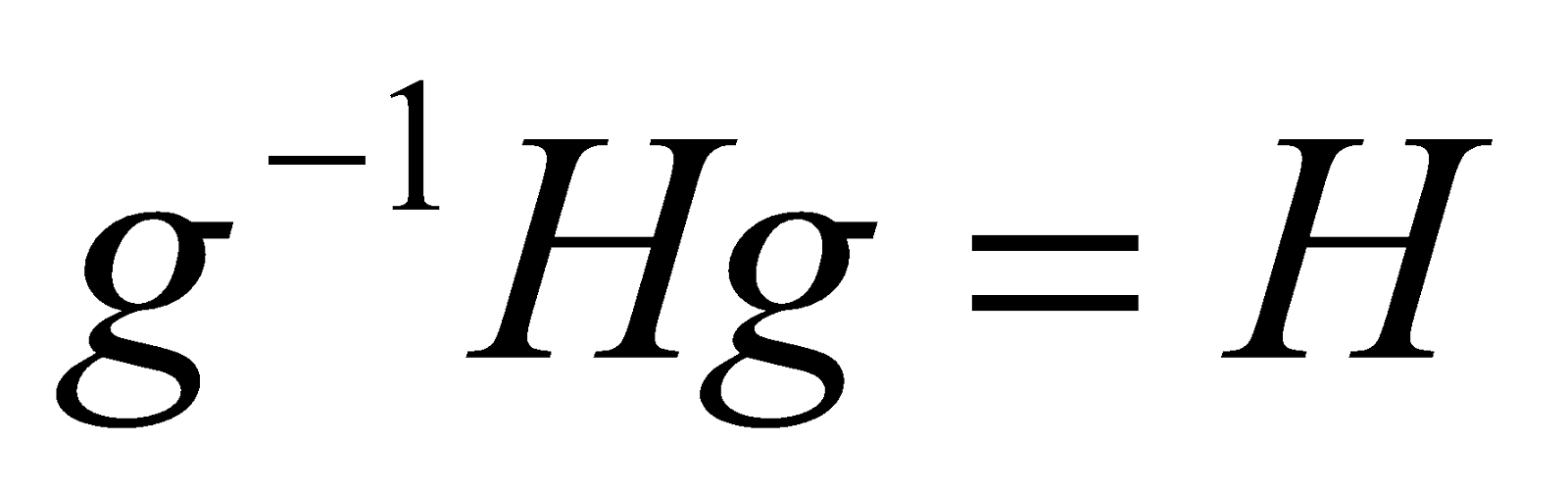for all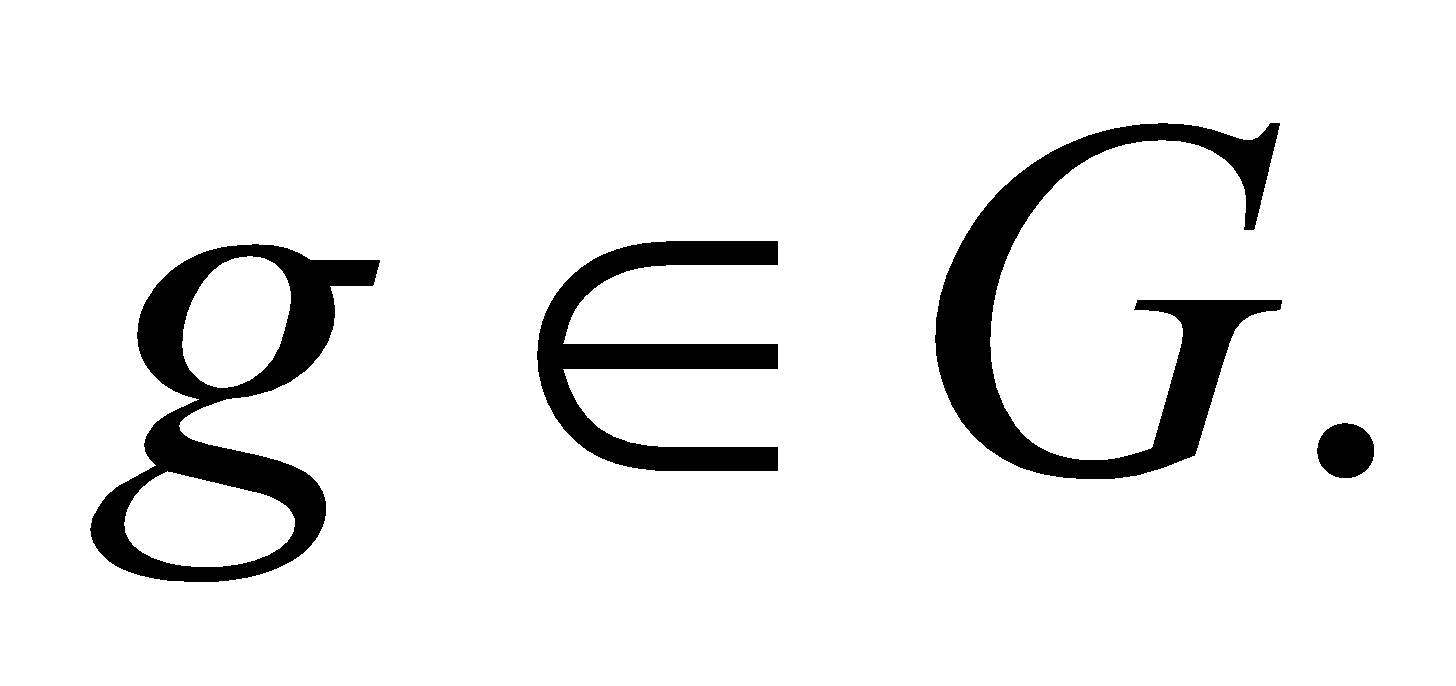4
9. Answer any two questions: 5x2=10
1. If G is a group such that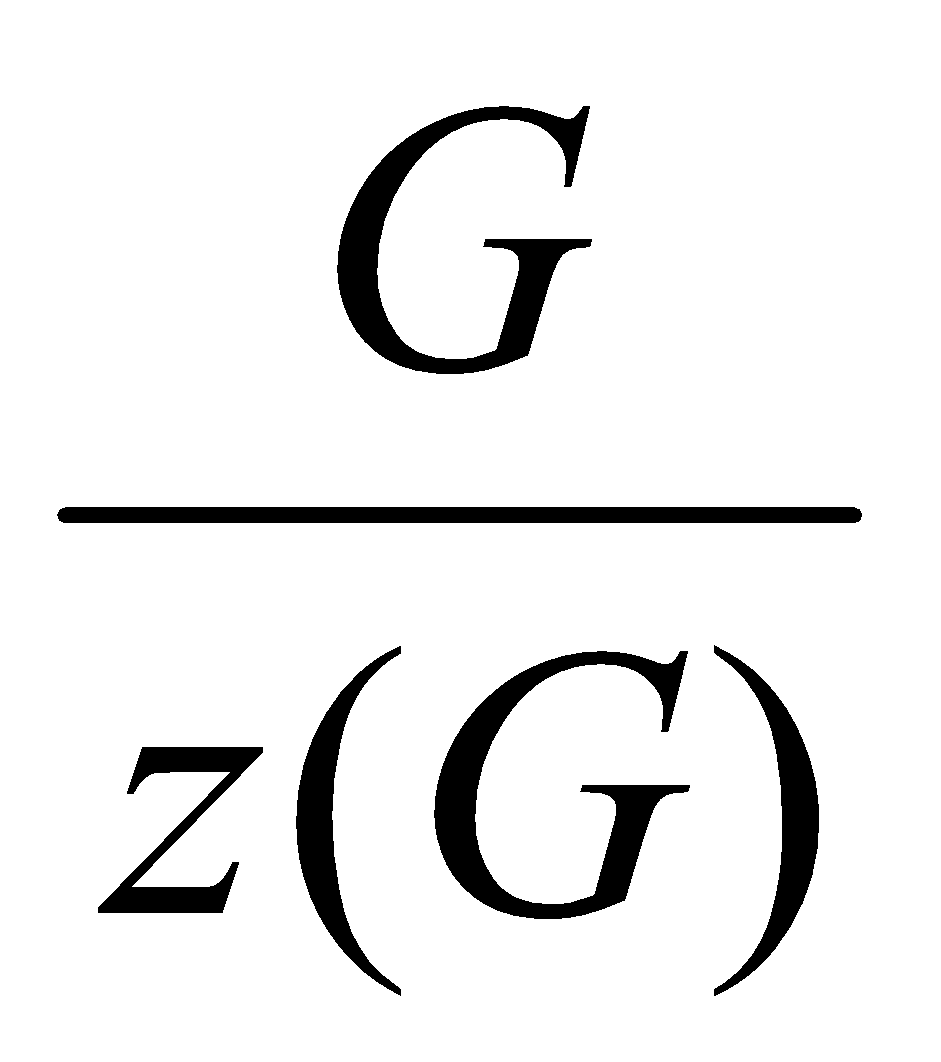is cyclic, where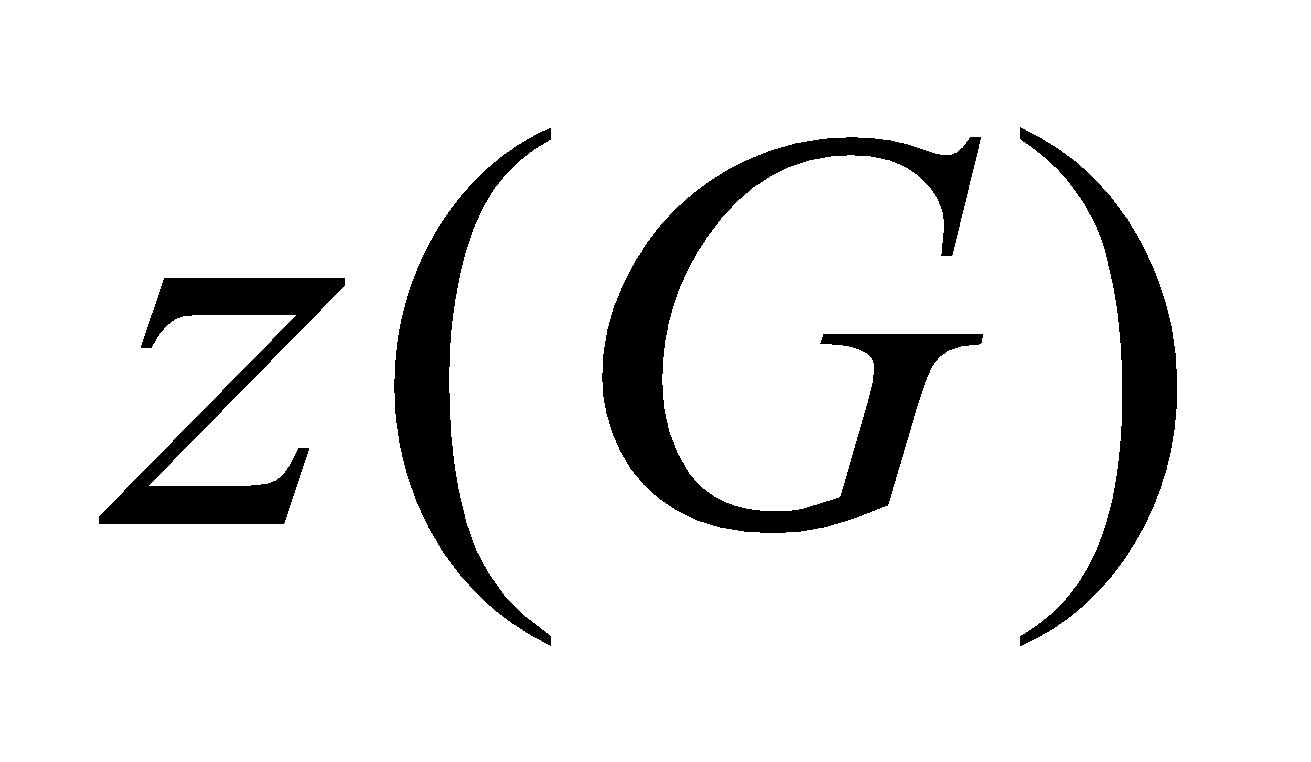is centre of G, then show that G is Abelian?
2. Prove that every quotient group of a cyclic group is cyclic.
3. State and prove fundamental theorem of group homomorphism.

***